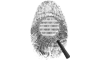## RSA Encryption

### STEP 1:

###### Select two prime numbers p and q
Prime Number 1(p):
Prime Number 2(q):

### STEP 4:

###### We will use Modular inverse function d = modinv(e,φ(n));
Prime Number 3(e):

### STEP 5 - Encryption:

###### encrypt(x) = xe mod n
Enter a char to encryption:

### STEP 6 - Decryption:

###### decrypt(x) = xd mod n
Enter encrypted text to decrypt: